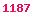# Regularization of the Diffusion Matrix through Multiple Euler Steps

Fries, Christian P.: A Short Note on the Regularization of the Diffusion Matrix for the Euler Scheme of an SDE. Version 0.5. 2006. [156 KB].

### Abstract

We consider a general Itô stochastic process

dX(t) = µ(t,X(t)) dt + σ(t,X(t)) • dW(t)

We show that the increment X(ti+1)-X(ti+1) of k Euler discretization steps of size h/k is equivalent to the increment X(ti+1)-X(ti+1) of one Euler step of step size h of an SDE with diffusion matrix (1,P1X)B,...,Pk-1X)B), where PkX) is a formal polynomial of order k and µX denotes the derivative of the drift µ(t,x) with respect to x. Depending on properties of the drift, this may result in a scheme corresponding to an SDE with full rank diffusion matrix.

It is natural to expect that successive Euler steps will result into a full rank diffusion since otherwise one may conclude that the model decouples into a diffusive part and a pure convectiouse part. The diffusive part could then be treated by the method presented while the convective part is treated by other methods. E.g. the method of characteristics (aka. method of lines).

### Classification

• MSC-class: 65C05 (Primary), 68U20, 60H35 (Secondary).
• ACM-class: G.3; I.6.8.
• JEL-class: C15, G13.
• Keywords: Monte-Carlo simulation, Euler scheme, singular diffusion matrix

Comments and suggestions welcomed at email@christian-fries.de.